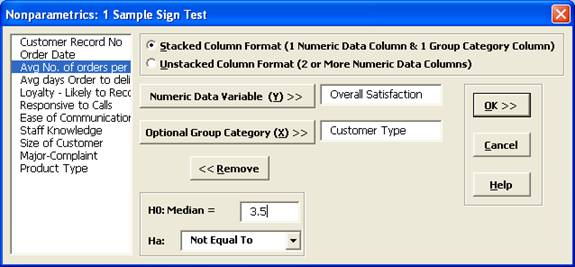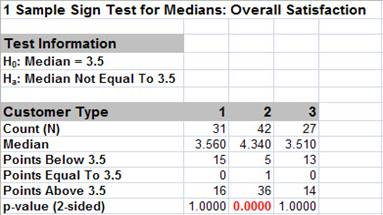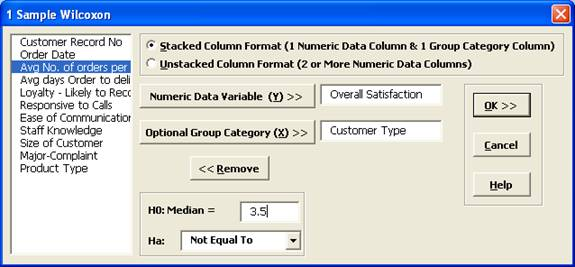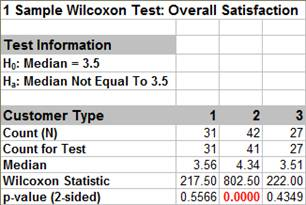Include Top

# How Do I Perform a One Sample Sign Test & One Sample Wilcoxon Signed Rank Test in Excel Using SigmaXL?

## One Sample Sign Test

The sign test is the simplest of the nonparametric tests, and is similar to testing if a two-sided coin is fair. Count the number of positive values (larger than hypothesized median), the number of negative values (smaller than the hypothesized median), and test whether there are significantly more positives (or negatives) than expected. The One Sample Sign Test is a nonparametric equivalent to the parametric One Sample t-Test.

Historically, our Median customer satisfaction score has been 3.5. We would like to see if this has changed, with the results grouped by customer type (H0: Median=3.5, Ha: Median≠3.5, α = 0.05).

1. Open Customer Data.xlsx, select Sheet 1 tab. Click SigmaXL > Statistical Tools > Nonparametric Tests>1 Sample Sign. If necessary, check Use Entire Data Table, click Next.

2. Ensure that Stacked Column Format is selected. Select Overall Satisfaction, click Numeric Data Variable (Y) >>; select Customer Type, click Optional Group Category (X) >>.

3. Enter 3.5 for the Null Hypothesis H0: Median value. Set Ha as Not Equal To.

4.5. Click OK. Results:

6.Note the P-Values. Customer Type 2 shows a significant change (increase) in Satisfaction Median (P-Value < .05), whereas Customer Types 1 and 3 show no change (P-Value ≥ .05). While the P-Values are not the same as those given by the 1 sample t-Test, the conclusions do match.

If Count (N) is less than or equal to 50, the Sign Test computes an exact P-Value using the binomial distribution. For N > 50, the P-Value is estimated using a normal approximation. Since this is always done automatically and is very fast, the Sign Test is not included in the separate Nonparametric Exact menu.

### One Sample Wilcoxon Signed Rank Test

The Wilcoxon Signed Rank test is a more powerful nonparametric test than the Sign Test, but it adds an assumption that the distribution of values is symmetric around the median. An example of a symmetric distribution is the uniform distribution. Symmetry can be observed with a histogram, or by checking to see if the Skewness is large (> .5 or < - .5).

The One Sample Wilcoxon Test is a nonparametric equivalent to the parametric One Sample t-Test.

Historically, our Median customer satisfaction score has been 3.5. We would like to see if this has changed, with the results grouped by customer type (H0: Median = 3.5, Ha: Median ≠ 3.5, α = 0.05).

1. Open Customer Data.xlsx, select Sheet 1 tab (or press F4 to activate last worksheet). Click SigmaXL > Statistical Tools > Nonparametric Tests > 1 Sample Wilcoxon. If necessary, check Use Entire Data Table, click Next.

2. Ensure that Stacked Column Format is selected. Select Overall Satisfaction, click Numeric Data Variable (Y) >>; select Customer Type, click Optional Group Category (X) >>.

3. Enter 3.5 for the Null Hypothesis H0:Median value. Keep Ha as Not Equal To.4. Click OK. Results:Note the p-values. Customer Type 2 shows a significant change (increase) in Satisfaction Median (p-value < .05), whereas Customer Types 1 and 3 show no change (p-value ≥ .05). Although the p-values are not identical to the sign test and t-Test, the conclusions match. (Note, in the case of Customer Type 2, the Sign Test is preferred since the data is not symmetrical but skewed).

# Web Demos

Our CTO and Co-Founder, John Noguera, regularly hosts free Web Demos featuring SigmaXL and DiscoverSim